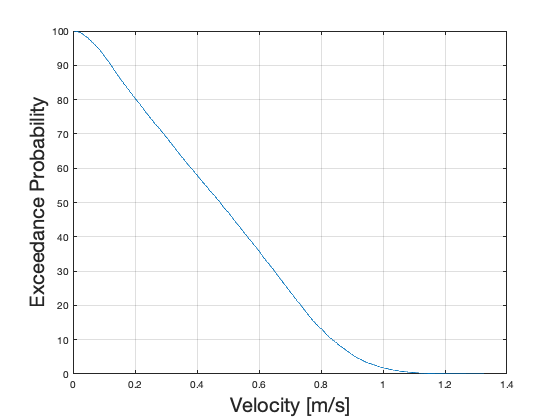# Example: MHKiT-MATLAB Tidal Module

The following example will familiarize the user with the MHKiT tidal module by stepping through the calculation of the velocity duration curve. The data file used in this example is stored in the \MHKiT\examples\data directory.

This example uses 1 year of data from the NOAA-Currents sites. A map of available currents stations is available at https://tidesandcurrents.noaa.gov/map/. The tidal io module includes two functions to import data: request_noaa_data which pulls data from the website, and read_noaa_json which loads a json file. The request function can save the json file for later use.
For simplicity, this example loads data from a json file into a MATLAB Series. This data contains 1 year of 6 minute averaged data from the Southampton Shoal Channel LB 6 (Station Number: s08010) in San Francisco Bay. The data includes 6 minute averaged direction [degrees] and speed [cm/s] indexed by time. The structure names returned by NOAA are 'd' for direction and 's' for speed. Since MHKIT uses SI units, speed is converted to m/s.
% Create a file name to save the requested data to
currents_file = "data/tidal/s08010.json"; % South Hampton Shoal LB
data = struct with fields:
id: "s08010" name: "Southampton Shoal Channel LB 6" lat: "37.9162" lon: "-122.4223" s: [18890×1 double] d: [18890×1 double] b: [18890×1 double] time: [1×18890 double]
% Example of how you could request NOAA-Currents data
%data = request_noaa_data("s08010","currents","20161101","20180401","write_json",currents_file);
% Convert discharge data from cm/s to m/s
data.s = data.s/100.;
The request_noaa_data has returned a Matlab Structure of time series data between November of 2016 and March of 2018. The time series data points are 6 minute averaged direction [degrees] and speed [cm/s] data indexed by time. The structure key names returned by NOAA are 'd' for direction and 's' for speed. The speed returned from NOAA is in cm/s so we converted this to m/s.

## Principal Flow Directions

An initial data check may look at the time series data to identify data gaps. To consider the velocity in one of the principal flow directions we apply the principal_flow_directions function. This function returns 2 directions (in degrees) corresponding to the flood and ebb directions of the tidal site. Principal flow directions are calculated based on the highest frequency directions. These directions are often close to 180 degrees apart, but are not required to be.
The plot_current_timeseries function plots velocity in either direction using the speed timeseries.
% We need to specify a histogram bin width for the directions to calculate
% the principal flow direction
width_direction = 1; % [degrees]
% Two principal directions are calculated based on the highest frequency
% direction
% One direction is the flood, the other is tide or ebb.
% they are 180 degrees apart, but not required to be
[direction1, direction2] = principal_flow_directions(data.d,width_direction);
% Set the flood and ebb directions based on site knowledge
flood = direction1 ; %flow into
ebb = direction2 ; % flow out
%plot the time series of current data using the flood direction
plot_current_timeseries(data,flood);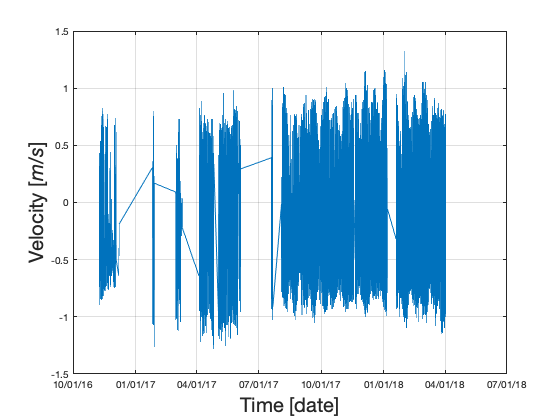The plot above shows that the NOAA site request did not return data for most of early and mid 2017. The IEC standard reccomends a minimum of 1 year of 10 minute averaged data (See IEC 201 for full description). For the purposes of demonstration this dataset is suffcient. To look at a speficic month we can slice the dataset before passing to the plotting function.
% Slice December of 2017 out of the full dataset
start = posixtime(datetime('2017-12-01','InputFormat','yyyy-MM-dd'));
stop = posixtime(datetime('2017-12-31','InputFormat','yyyy-MM-dd'));
index = data.time > start & data.time < stop ;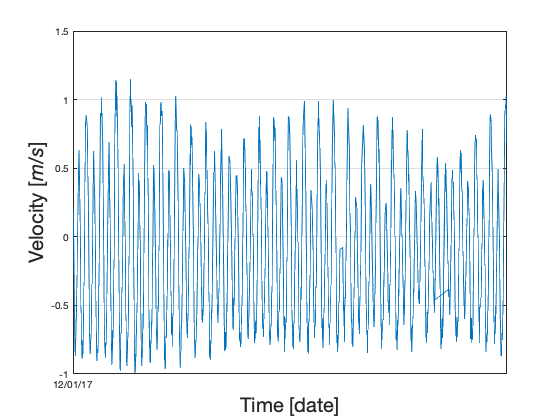## Joint Probability Distribution

Direction and velocity can be viewed as a joint probability distribution on a polar plot. This plot helps visually show the flood and ebb directions and the frequency of particular directional velocities.
% Set the joint probability bit widths
width_direction = 1 ; %[degrees]
width_velocity = 0.1 ; %[m/s]
% Plot the joint probability distribution
figure;
plot_joint_probability_distribution(data,width_direction,width_velocity);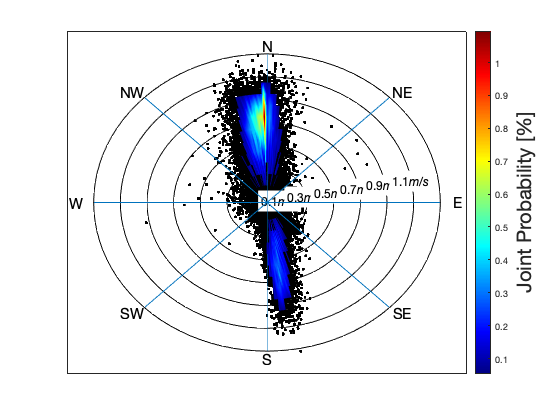## Rose Plot

A rose plot shows the same information as the joint probability distribution but the probability is now the r-axis, and the velocity is the contour value. As compared to a joint probability distribution plot, a rose plot can be more readable when using larger bins sizes.
% A rose plot is more readable using larger bin sizes than the jpd plot
width_direction = 10; % [degrees]
width_velocity = 0.25; %[m/s]
%plot the rose plot
%f2= figure;
figure;
plot_rose(data,width_direction,width_velocity,"flood_ebb",[flood,ebb]);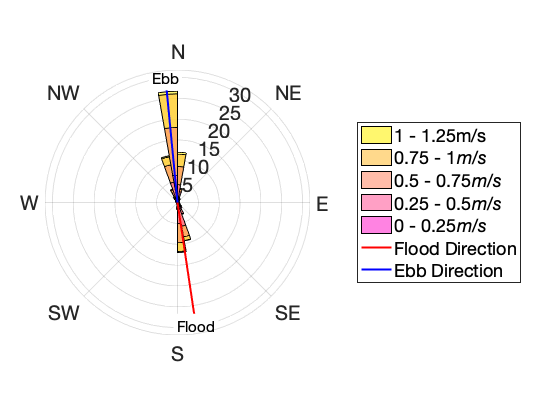## Velocity Duration Curve

The velocity duration curve shows the probability of achieving a particular velocity value. After computing the exceedance probability, the rank order of velocity values can be plotted as follows.
% Calculate teh exceedance probability of the data
data.Discharge = data.s; % need to change structure name for exceedance probability function
F = exceedance_probability(data);
% Plot the VDC
plot_velocity_duration_curve(data.s,F.F);